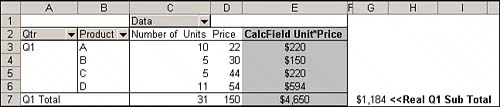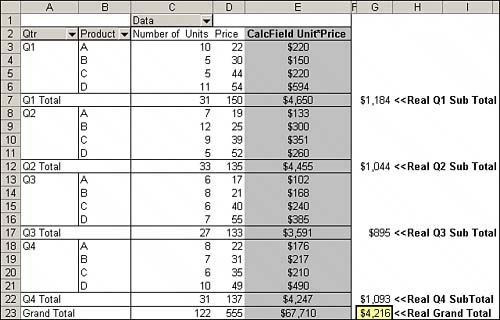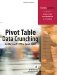# Rules and Shortcomings of Pivot Table Calculations

Although there is no better way to integrate your calculations into a pivot table than using calculated fields and calculated items, they do come with their own set of drawbacks. It's important you understand what goes on behind the scenes when you use pivot table calculations, and even more important to be aware of the boundaries and limitations of calculated fields and calculated items in order to avoid potential errors in your data analysis.

This section will highlight the rules concerning calculated fields and calculated items that you will most likely encounter when working with pivot table calculations.

#### Order of Operator Precedence

Calculations in pivot tables follow the order of operator precedencethat is, the defined order that Excel evaluates and performs calculations. It is important to understand the order of operations in Excel in order to avoid miscalculating your data.

Just as in a spreadsheet, you can use any operator in your calculation formulas, meaning any symbol that represents a calculation to perform (+, -, *, /, %, ^, and so on). When you perform a calculation that combines several operators, as in (2+3)*4/50%, Excel evaluates and performs the calculation in a specific order. The order of operations for Excel is as follows:

• Evaluate items in parentheses.

• Evaluate ranges (:).

• Evaluate intersections (spaces).

• Evaluate unions (,).

• Perform negation (-).

• Convert percentages (%). (For example, turn 50% to .50.)

• Perform exponentiation (^).

• Perform multiplication (*) and division (/). These operations are of equal precedence.

• Perform addition (+) and subtraction (-). These operations are of equal precedence.

• Evaluate text operator (&). This is known as concatenation.

• Perform comparisons (=, <>, <=, >=).

• Finally, operations that are equal in precedence will be performed from left to right.

Consider this basic example: The correct answer to (2+3)*4 is 20. However, if you leave out the parentheses, as in 2+3*4, Excel will perform the calculation like this:

3*4 = 12 + 2 = 14

The order of operator precedence mandates that Excel perform multiplication before subtraction. Entering 2+3*4 will give you the wrong answer. Because Excel evaluates and performs all calculations in parentheses first, placing 2+3 inside parentheses ensures the correct answer.

Here is another widely demonstrated example: If you enter 10^2, which represents the exponent 10 to the 2nd power, as a formula, Excel will return 100 as the answer. If you enter -10^2, you would expect -100 to be the result. Instead, Excel will return 100 yet again. The reason is that Excel performs negation before exponentiation, meaning Excel is converting 10 to -10 before the exponentiation, effectively calculating -10*-10, which indeed equals 100. Using parentheses in the formula, (10^2), will ensure that Excel calculates the exponent before negating the answer, giving you -100.

#### Cell References and Named Ranges

You cannot use cell references or named ranges in your calculation formulas, because when you create calculations in a pivot table, you are essentially working in a vacuum. The only data available to you is the data that exists in the pivot cache. Therefore, you cannot reach outside the confines of the pivot cache to reference cells or named ranges in your formula.

#### Worksheet Functions

You can use any worksheet function that does not require cell references or defined names as an argument. In effect, this means you can use any worksheet function that does not require cell references or defined names to work. Some of the many functions that fall into this category include COUNT, AVERAGE, IF, AND, NOT, and OR.

#### Constants

You may use any constant in your pivot table calculations. Constants are static values that do not change. For example, 5 is a constant in the following formula:

[Units Sold]*5

Though the value of Units Sold may change based on the available data, 5 will always have the same value.

#### Referencing Totals

Your calculation formulas cannot reference a pivot table's subtotals or grand total. In other words, you cannot use the result of a subtotal or grand total as a variable or argument in your calculated field.

#### Rules Specific to Calculated Fields

Calculated field calculations are always performed against totals as opposed to individual data items. In basic terms, Excel will always calculate data fields, subtotals, and grand totals before evaluating your calculated field. This means that your calculated field is always applied to the sum of the underlying data.

The example shown in Figure 6.30 demonstrates how this can adversely affect your data analysis.

##### Figure 6.30. Although the calculated field is correct for the individual data items in your pivot table, the subtotal is mathematically incorrect.In each quarter, you need to get the total revenue for every product by multiplying the number of units sold by the price. If you look at Q1 first, you will immediately see the problem. Instead of returning the sum of 220+150+220+594, which would give you \$1,184, the subtotal is multiplying the sum of number of units by the sum of the price, which returns the wrong answer.

As you can see in Figure 6.31, including the whole year in your analysis compounds the problem.

##### Figure 6.31. The grand total for the year as a whole is completely wrong.Unfortunately, there is no solution to this problem, but there is a workaround. In worst-case scenarios, you can configure your settings to eliminate subtotals and grand totals and then calculate your own totals. Figure 6.32 shows an example of this scenario.

##### Figure 6.32. Calculating your own totals can avoid reporting incorrect data.#### Rules Specific to Calculated Items

You cannot use calculated items in a pivot table that uses averages, standard deviations, or variances. Conversely, you cannot use averages, standard deviations, or variances in a pivot table that contains a calculated item.

You cannot use a page field to create a calculated item, nor can you move any calculated item to the page area.

You cannot add a calculated item to a report that has a grouped field, nor can you group any field in a pivot table that contains a calculated item.

When building your calculated item formula, you cannot reference items from a field other than the one you are working with.

As you think about the section you have just read, don't be put off by these shortcomings. Despite the clear limitations highlighted, the ability to create custom calculations directly into your pivot table remains a powerful and practical feature that can enhance your data analysis. Now that you are aware of the inner workings of pivot table calculations and understand the limitations of calculated fields and items, you can avoid the pitfalls and use these features with confidence.Pivot Table Data Crunching for Microsoft Office Excel 2007
ISBN: 0789736012
EAN: 2147483647
Year: 2003
Pages: 140

Similar book on Amazon